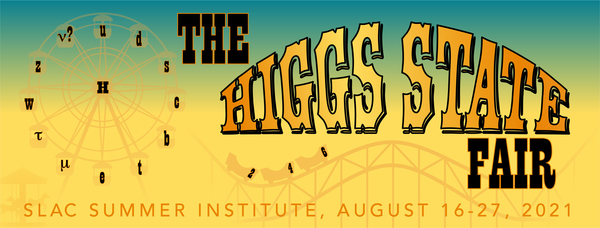#49th SLAC Summer Institute (SSI 2021)

16-27 August 2021
SLAC
America/Los_Angeles timezone

## Test of the Realism in Particle Physics

Not scheduled
20m
ZOOM videoconf (SLAC)

### Speaker

Ms ANNA EFIMOVA (M.V. Lomonosov Moscow State University)

### Description

The Realism , which is based on the properties of classical objects can be violated in quantum world. Assumption that observables of the system can jointly exist (Classical realism) and Locality can be wrong in Quantum Mechanics .

Test of the Realism can be done with Wigner inequalities . There should be three dichotomous variables in Wigner inequalities and these inequalities consist of probabilities of detecting correlated properties between pairs of entangled particles. As dichotomous variables neutral pseudoscalar mesons  (states with certain flavour, states with certain CP-parity and states with certain mass and lifetime) can be considered.

In this work theoretical proof of the violations of 3-time-dependent Wigner inequalities :

\begin{eqnarray}
&\le& w\left(a_+^{(2)},c_+^{(1)},t_0\right)\cdot w\left(a_+^{(2)}(t_0)\to a_+^{(2)}(t_2)\right)\cdot\nonumber\
&\cdot&\left (w\left(b_+^{(1)}(t_0)\to b_+^{(1)}(t_1)\right)+w\left(b_-^{(1)}(t_0)\to b_+^{(1)}(t_1)\right)\right)+ \nonumber
\
&+& w\left(a_-^{(2)},c_+^{(1)},t_0\right)\cdot w\left(a_-^{(2)}(t_0)\to a_+^{(2)}(t_2)\right)\cdot\nonumber\
&\cdot&\left(w\left(b_+^{(1)}(t_0)\to b_+^{(1)}(t_1)\right)+w\left(b_-^{(1)}(t_0)\to b_+^{(1)}(t_1)\right)\right) + \nonumber
\
&+& w\left(c_+^{(2)},b_+^{(1)},t_0\right)\cdot w\left(b_+^{(1)}(t_0)\to b_+^{(1)}(t_!)\right)\cdot\nonumber\
&\cdot&\left(w\left(a_+^{(2)}(t_0)\to a_+^{(2)}(t_2)\right)+w\left(a_-^{(2)}(t_0)\to a_+^{(2)}(t_2)\right)\right) + \nonumber
\
&+&w\left(c_+^{(2)},b_-^{(1)},t_0\right)\cdot w\left(b_-^{(1)}(t_0)\to b_+^{(1)}(t_1)\right)\cdot\nonumber\
&\cdot&\left(w\left(a_+^{(2)}(t_0)\to a_+^{(2)}(t_2)\right)+w\left(a_-^{(2)}(t_0)\to a_+^{(2)}(t_2)\right)\right), \nonumber
\end{eqnarray}
in systems of $K^0$, $B_s$, $D^0$- mesons  is shown. The inequalities is tested for the Bell pure state $|\Psi^->$:
$$|\Psi^->=\dfrac{1}{\sqrt{2}}\left(|M^{(2)}>|\bar{M}^{(1)}>-|\bar{M}^{(2)}>|M^{(1)}>\right),$$ where $M$- a certain meson, and also for Werner state, where background is modeled by identity matrix. Areas of violation depending on $t_1$ and $t_2$ of this inequality were found in systems of neutral pseudoscalar mesons. Also it was shown that for experimental testing time-dependent Wigner inequalities are more prospective than time-independent Wigner inequalities.
 N. Nikitin, K. Toms, Phys. Rev. D 95, 052103 (2017).
 A. Einstein, B. Podolsky and N. Rosen, Phys. Rev. 47, 777 (1935).
 E. P. Wigner, Am. J. Phys. 38, 1005 (1970).
 F. Uchiyama, Phys. Lett. A 231, 295 (1997) .
 A. Efimova, J. of Phys.: Conf. Ser. 1439 012008 (2020).

### Primary author

Ms ANNA EFIMOVA (M.V. Lomonosov Moscow State University)

### Presentation Materials

 Anna_Efimova_Poster.pdf Anna_Efimova_video.mp4